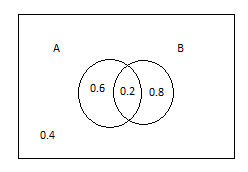QuestionAnswers

# If a Venn diagram used to represent probabilities, sample space of events is represented byA. SquareB. TriangleC. CircleD. Rectangle

Hint: A diagram that shows all possible logical relations between finite collections of different sets are known as Venn diagram. It is also known as primary diagram, logical diagram or the set diagram. A Venn diagram is represented by overlapping circles within a rectangle which contains all elements so the set. Probability can be found using Venn diagram of three events by simply finding the sum of individual probabilities as $P\left( {X{\text{ }}or{\text{ }}Y{\text{ }}or{\text{ }}Z} \right){\text{ }} = {\text{ }}P\left( X \right) + P\left( Y \right) + P\left( Z \right)$Where sum of all values of diagram is $0.6 + 0.2 + 0.8 + 0.4 = 2.0$